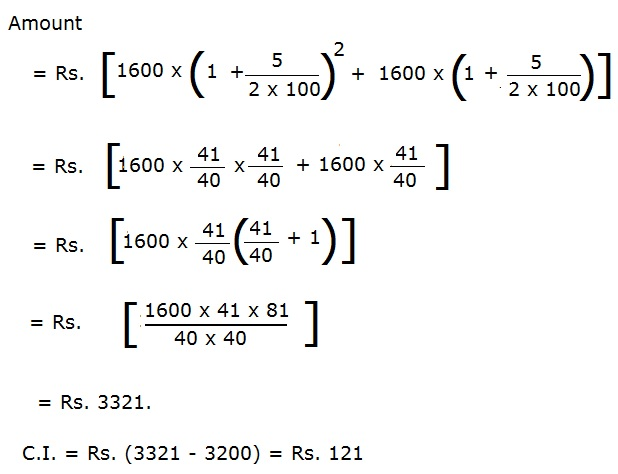# What is Compound Interest?

### What is ‘Compound Interest’

Compound interest is interest calculated on the initial principal and also on the accumulated interest of previous periods of a deposit or loan. Compound interest can be thought of as “interest on interest,” and will make a deposit or loan grow at a faster rate than simple interest, which is interest calculated only on the principal amount. The rate at which compound interest accrues depends on the frequency of compounding; the higher the number of compounding periods, the greater the compound interest. Thus, the amount of compound interest accrued on \$100 compounded at 10% annually will be lower than that on \$100 compounded at 5% semi-annually over the same time period. Compound interest is also known as compounding.

#### Question:

A bank offers 5% compound interest calculated on half-yearly basis. A customer deposits Rs. 1600 each on 1st January and 1st July of a year. At the end of the year, the amount he would have gained by way of interest is:

A. Rs. 120

B. Rs. 121

C. Rs. 122

D. Rs. 123

#### Explanation: